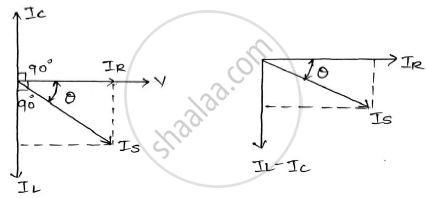# In an R-l-c Parallel Circuit the Current Through the Resistor, Inductor(Pure), and Capacitor (Pure) Are 20a, 15a and 40a Respectively. What is the Current from the Supply ?Draw the Phasor Diagram. - Basic Electrical and Electronics Engineering

Sum

In an R-L-C parallel circuit the current through the resistor, inductor(pure), and capacitor (pure) are 20A, 15A and 40A respectively. What is the current from the supply ?draw the phasor diagram.

#### Solution

"I"_"R"=20"A"        "I"_"L"=15"A"      "I"_"C"=40"A"

IS be the source current.

"I"_"S"^2="I"_"R"^2+("I"_"L"-"I"_"C")^2

"I"_"S"=sqrt("I"_"R"^2+("I"_"L"-"I"_"C")^2)

"I"_"S"=sqrt(20^2+(15-40)^2)=sqrt(20^2+(-25)^2

IS= 𝟑𝟐. 𝟎𝟏𝟓 AConcept: R-L, R-C and R-LC parallel circuits
Is there an error in this question or solution?